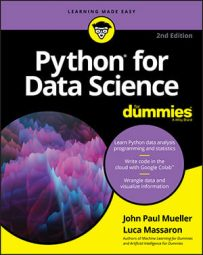##### Python for Data Science For DummiesScikit-learn is a focal point for data science work with Python, so it pays to know which methods you need most. The following table provides a brief overview of the most important methods used for data analysis.
 Syntax Usage Description `model_selection.cross_val_score` Cross-validation phase Estimate the cross-validation score `model_selection.KFold` Cross-validation phase Divide the dataset into k folds for cross validation `model_selection.StratifiedKFold` Cross-validation phase Stratified validation that takes into account the distribution of the classes you predict `model_selection.train_test_split` Cross-validation phase Split your data into training and test sets `decomposition.PCA` Dimensionality reduction Principal component analysis (PCA) `decomposition.RandomizedPCA` Dimensionality reduction Principal component analysis (PCA) using randomized SVD `feature_extraction.FeatureHasher` Preparing your data The hashing trick, allowing you to accommodate a large number of features in your dataset `feature_extraction.text.CountVectorizer` Preparing your data Convert text documents into a matrix of count data `feature_extraction.text.HashingVectorizer` Preparing your data Directly convert your text using the hashing trick `feature_extraction.text.TfidfVectorizer` Preparing your data Creates a dataset of TF-IDF features `feature_selection.RFECV` Feature selection Automatic feature selection `model_selection.GridSearchCV` Optimization Exhaustive search in order to maximize a machine learning algorithm `linear_model.LinearRegression` Prediction Linear regression `linear_model.LogisticRegression` Prediction Linear logistic regression `metrics.accuracy_score` Solution evaluation Accuracy classification score `metrics.f1_score` Solution evaluation Compute the F1 score, balancing accuracy and recall `metrics.mean_absolute_error` Solution evaluation Mean absolute error regression error `metrics.mean_squared_error` Solution evaluation Mean squared error regression error `metrics.roc_auc_score` Solution evaluation Compute Area Under the Curve (AUC) from prediction scores `naive_bayes.MultinomialNB` Prediction Multinomial Naïve Bayes `neighbors.KNeighborsClassifier` Prediction K-Neighbors classification `preprocessing.Binarizer` Preparing your data Create binary variables (feature values to 0 or 1) `preprocessing.Imputer` Preparing your data Missing values imputation `preprocessing.MinMaxScaler` Preparing your data Create variables bound by a minimum and maximum value `preprocessing.OneHotEncoder` Preparing your data Transform categorical integer features into binary ones `preprocessing.StandardScaler` Preparing your data Variable standardization by removing the mean and scaling to unit variance